# Bounded Model Checking A Biere A Cimatti E

• Slides: 30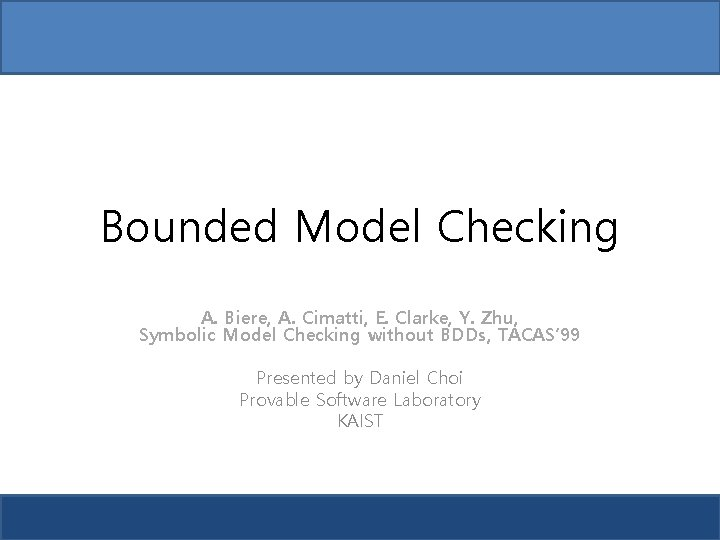Bounded Model Checking A. Biere, A. Cimatti, E. Clarke, Y. Zhu, Symbolic Model Checking without BDDs, TACAS’ 99 Presented by Daniel Choi Provable Software Laboratory KAIST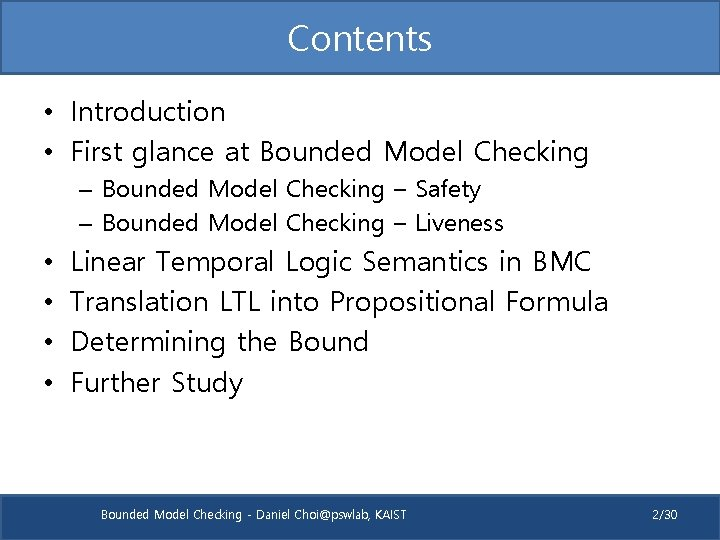Contents • Introduction • First glance at Bounded Model Checking – Safety – Bounded Model Checking – Liveness • • Linear Temporal Logic Semantics in BMC Translation LTL into Propositional Formula Determining the Bound Further Study Bounded Model Checking - Daniel [email protected], KAIST 2/30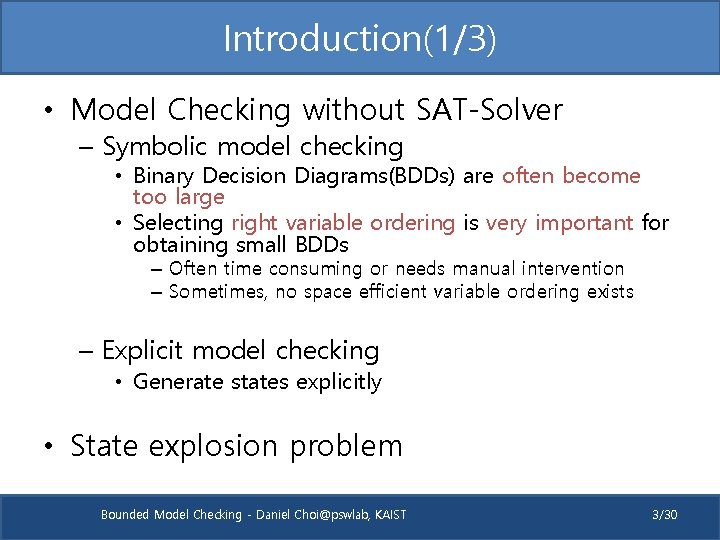Introduction(1/3) • Model Checking without SAT-Solver – Symbolic model checking • Binary Decision Diagrams(BDDs) are often become too large • Selecting right variable ordering is very important for obtaining small BDDs – Often time consuming or needs manual intervention – Sometimes, no space efficient variable ordering exists – Explicit model checking • Generate states explicitly • State explosion problem Bounded Model Checking - Daniel [email protected], KAIST 3/30Introduction(2/3) • Variable ordering of BDDs – BDD of (a 1 ∧ b 1) ∨ (a 2 ∧ b 2) a 1 Bad ordering a 1 Good ordering b 1 a 2 b 1 b 2 0 1 Bounded Model Checking - Daniel [email protected], KAIST 0 1 4/30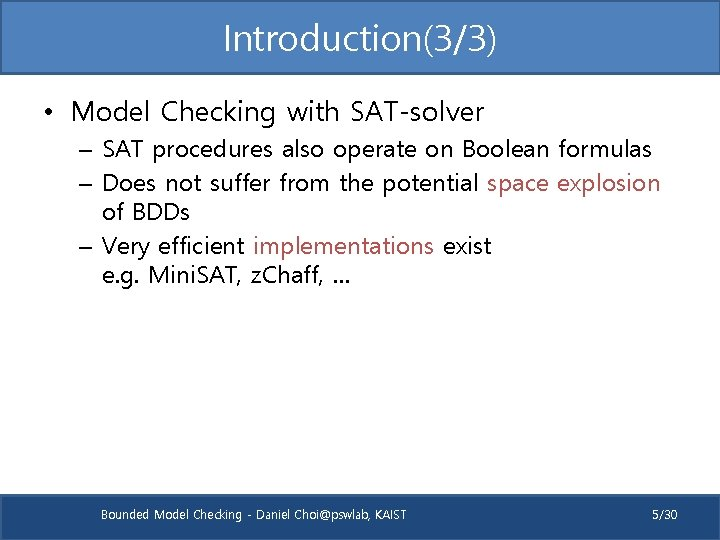Introduction(3/3) • Model Checking with SAT-solver – SAT procedures also operate on Boolean formulas – Does not suffer from the potential space explosion of BDDs – Very efficient implementations exist e. g. Mini. SAT, z. Chaff, … Bounded Model Checking - Daniel [email protected], KAIST 5/30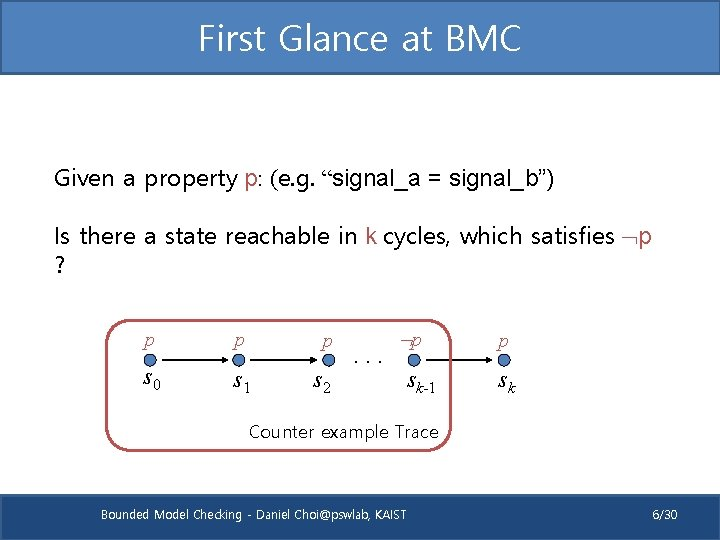First Glance at BMC Given a property p: (e. g. “signal_a = signal_b”) Is there a state reachable in k cycles, which satisfies p ? p p p s 0 s 1 s 2 . . . p sk-1 p sk Counter example Trace Bounded Model Checking - Daniel [email protected], KAIST 6/30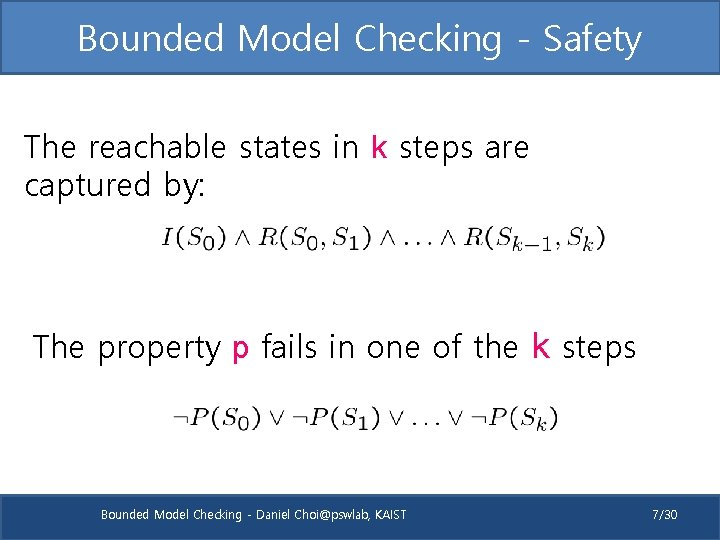Bounded Model Checking - Safety The reachable states in k steps are captured by: The property p fails in one of the k steps Bounded Model Checking - Daniel [email protected], KAIST 7/30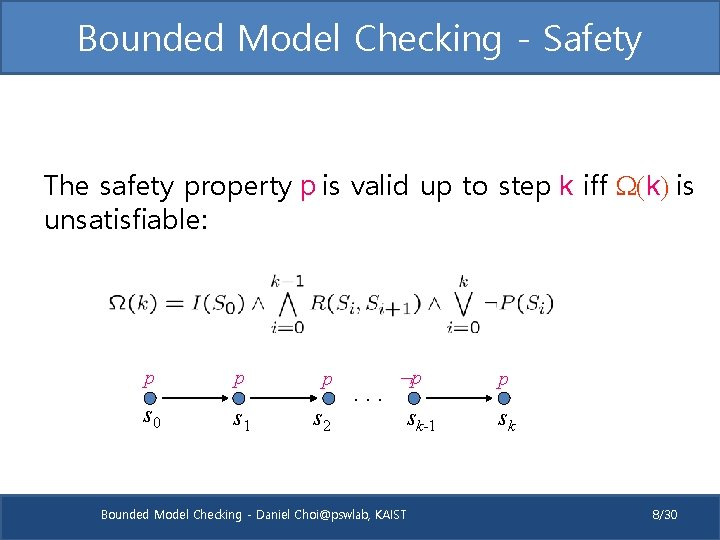Bounded Model Checking - Safety The safety property p is valid up to step k iff W(k) is unsatisfiable: p p p s 0 s 1 s 2 . . . p Bounded Model Checking - Daniel [email protected], KAIST sk-1 p sk 8/30Bounded Model Checking - Safety Example: a two bit counter Initial state: I: l ^ r 00 11 01 10 Transition: R: l’ = (l r) ^ r’ = r Property: G ( l r). For k = 2, W(k) is unsatisfiable. For k = 3 W(k) is satisfiable Bounded Model Checking - Daniel [email protected], KAIST 9/30Bounded Model Checking - Liveness There is no counterexample of length k to the Liveness property Fp iff W(k) is unsatisfiable: Loop Constraint = : p s 0 : p s 1 : p s 2 . . . p Bounded Model Checking - Daniel [email protected], KAIST sk-1 : p sk 10/30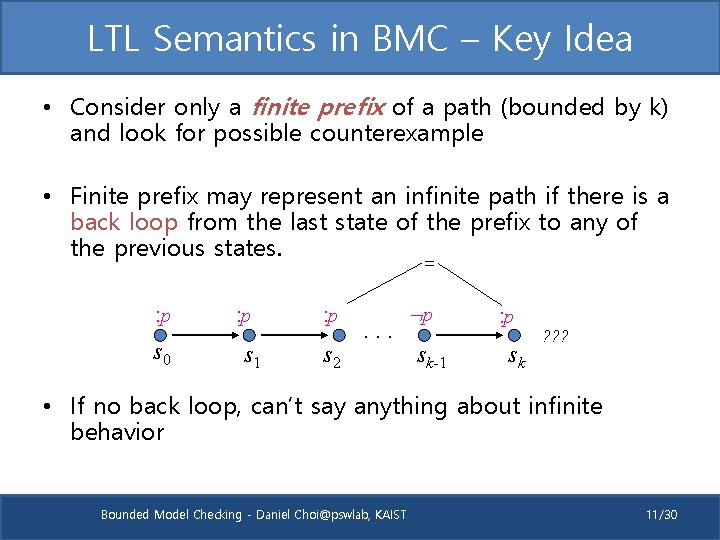LTL Semantics in BMC – Key Idea • Consider only a finite prefix of a path (bounded by k) and look for possible counterexample • Finite prefix may represent an infinite path if there is a back loop from the last state of the prefix to any of the previous states. = : p s 0 : p s 1 : p s 2 . . . p sk-1 : p sk ? ? ? • If no back loop, can’t say anything about infinite behavior Bounded Model Checking - Daniel [email protected], KAIST 11/30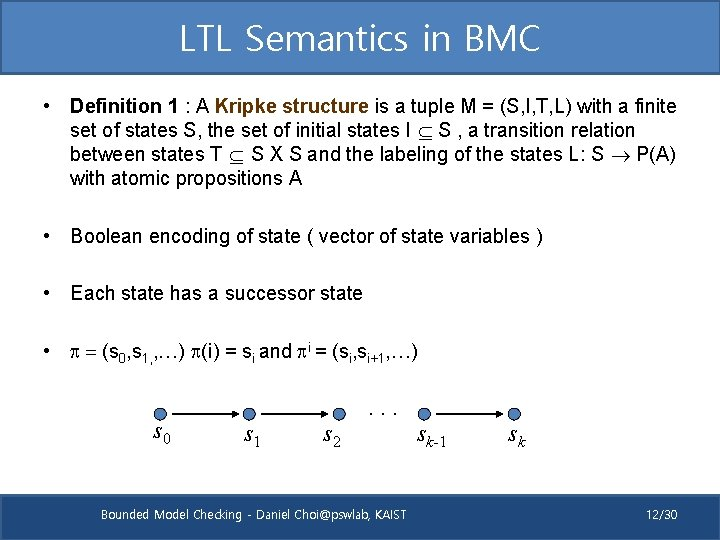LTL Semantics in BMC • Definition 1 : A Kripke structure is a tuple M = (S, I, T, L) with a finite set of states S, the set of initial states I S , a transition relation between states T S X S and the labeling of the states L: S P(A) with atomic propositions A • Boolean encoding of state ( vector of state variables ) • Each state has a successor state • p = (s 0, s 1, , …) p(i) = si and pi = (si, si+1, …) s 0 s 1 s 2 . . . Bounded Model Checking - Daniel [email protected], KAIST sk-1 sk 12/30LTL Semantics • Definition 2 (Semantics of LTL) : Let M be a Kripke structure, p be a path in M and f be an LTL formula. Then p ⊨ f ( f is valid along p) is defined as Bounded Model Checking - Daniel [email protected], KAIST 13/30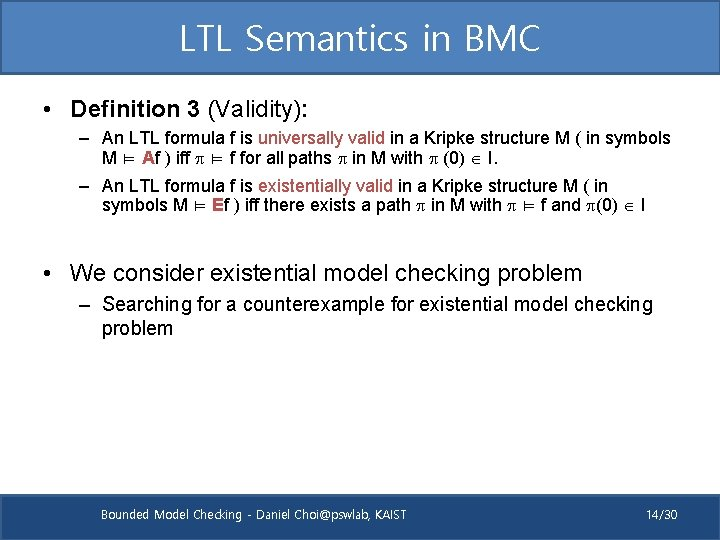LTL Semantics in BMC • Definition 3 (Validity): – An LTL formula f is universally valid in a Kripke structure M ( in symbols M ⊨ Af ) iff p ⊨ f for all paths p in M with p (0) I. – An LTL formula f is existentially valid in a Kripke structure M ( in symbols M ⊨ Ef ) iff there exists a path p in M with p ⊨ f and p(0) I • We consider existential model checking problem – Searching for a counterexample for existential model checking problem Bounded Model Checking - Daniel [email protected], KAIST 14/30LTL Semantics in BMC • However, we are considering bounded sequence … • Definition 4 : For l k we call a path p a (k, l)-loop if p(k) p(l) and p =u. vw with u = (p(0), …. , p(l-1)) and v=(p(l), . . , p(k)). We call p simply a k-loop if there is an l N with l Mk for which p is a (k, l)-loop Bounded Model Checking - Daniel [email protected], KAIST 15/30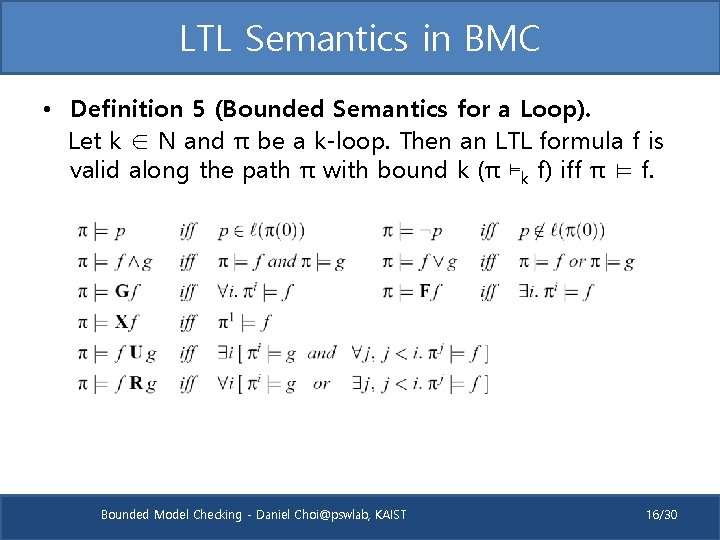LTL Semantics in BMC • Definition 5 (Bounded Semantics for a Loop). Let k ∈ N and π be a k-loop. Then an LTL formula f is valid along the path π with bound k (π ⊨k f) iff π ⊨ f. Bounded Model Checking - Daniel [email protected], KAIST 16/30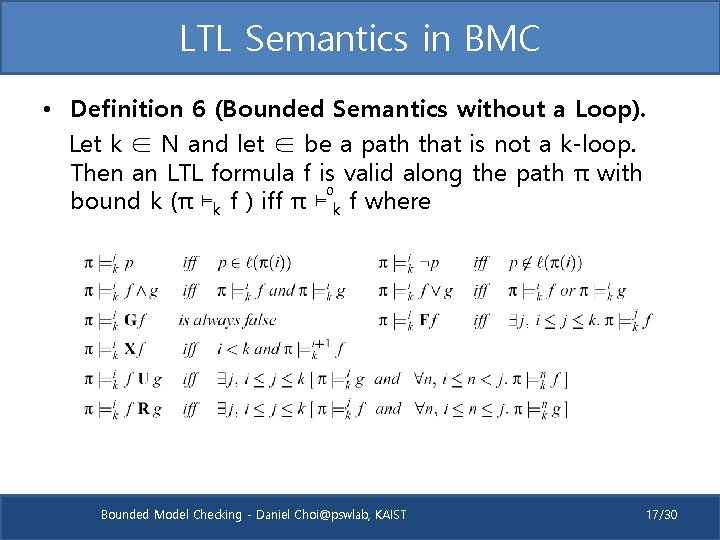LTL Semantics in BMC • Definition 6 (Bounded Semantics without a Loop). Let k ∈ N and let ∈ be a path that is not a k-loop. Then an LTL formula f is valid along the path π with 0 bound k (π ⊨k f ) iff π ⊨ k f where Bounded Model Checking - Daniel [email protected], KAIST 17/30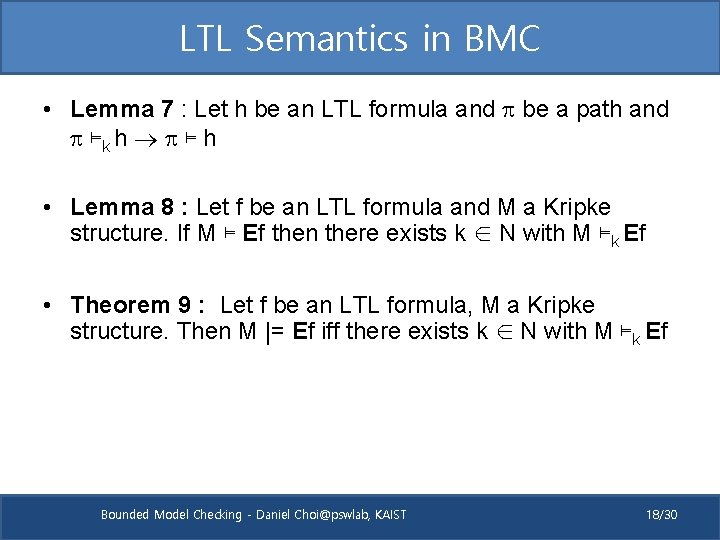LTL Semantics in BMC • Lemma 7 : Let h be an LTL formula and p be a path and p ⊨k h p ⊨ h • Lemma 8 : Let f be an LTL formula and M a Kripke structure. If M ⊨ Ef then there exists k ∈ N with M ⊨k Ef • Theorem 9 : Let f be an LTL formula, M a Kripke structure. Then M |= Ef iff there exists k ∈ N with M ⊨k Ef Bounded Model Checking - Daniel [email protected], KAIST 18/30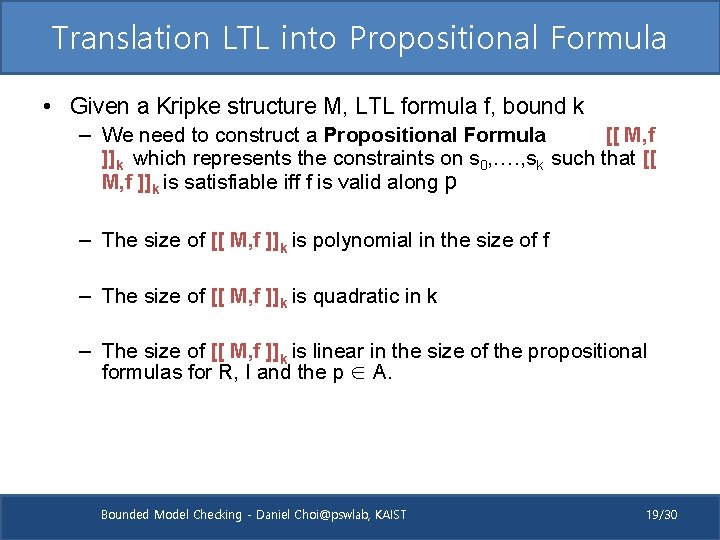Translation LTL into Propositional Formula • Given a Kripke structure M, LTL formula f, bound k – We need to construct a Propositional Formula [[ M, f ]]k which represents the constraints on s 0, …. , sk such that [[ M, f ]]k is satisfiable iff f is valid along p – The size of [[ M, f ]]k is polynomial in the size of f – The size of [[ M, f ]]k is quadratic in k – The size of [[ M, f ]]k is linear in the size of the propositional formulas for R, I and the p ∈ A. Bounded Model Checking - Daniel [email protected], KAIST 19/30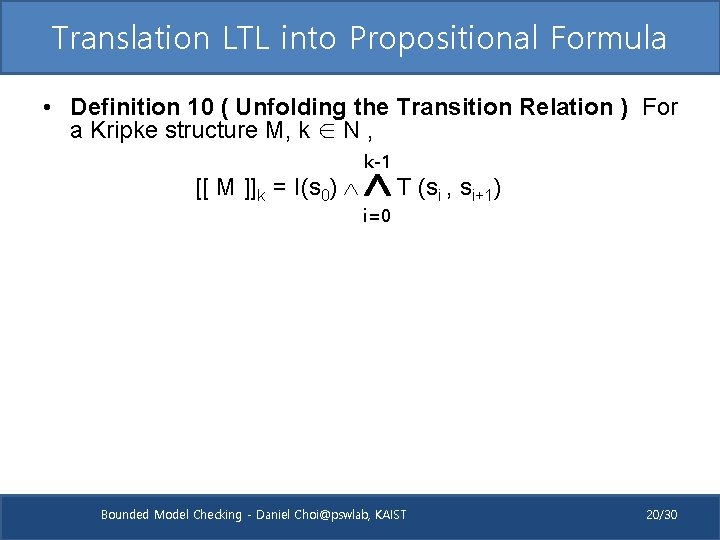Translation LTL into Propositional Formula • Definition 10 ( Unfolding the Transition Relation ) For a Kripke structure M, k ∈ N , [[ M ]]k = I(s 0) k-1 T (s , s i=0 Bounded Model Checking - Daniel [email protected], KAIST i i+1) 20/30Example – 3 bit shift register • 3 -bit misbehaving shift register (x, x, x) • T(x, x’): (x’=x) (x’=x) (x’=1) • “Eventually register will be empty” : AF( x=0 ) – AF( x=0 ) ¬EG( x != 0 ) • Restrict search to path having k+1 states (k=2) x 0 x 1 x 2 x 0 x 1 x 2 x 0 x 1 x 2 x 0 x 1 x 2 Bounded Model Checking - Daniel [email protected], KAIST 21/30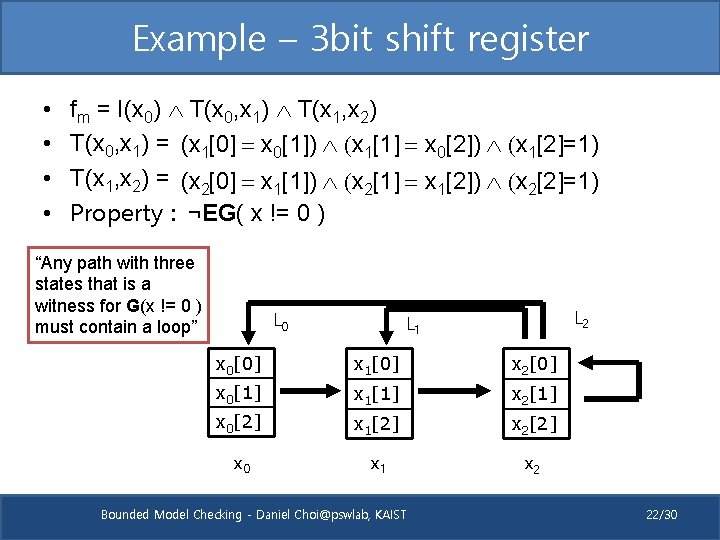Example – 3 bit shift register • • fm = I(x 0) T(x 0, x 1) T(x 1, x 2) T(x 0, x 1) = (x 1 = x 0) (x 1 = x 0) (x 1=1) T(x 1, x 2) = (x 2 = x 1) (x 2 = x 1) (x 2=1) Property : ¬EG( x != 0 ) “Any path with three states that is a witness for G(x != 0 ) must contain a loop” L 0 L 2 L 1 x 0 x 1 x 2 x 0 x 1 x 2 x 0 x 1 x 2 x 0 x 1 x 2 Bounded Model Checking - Daniel [email protected], KAIST 22/30Translation LTL into Propositional Formula • Definition 10 ( Unfolding the Transition Relation ) For a Kripke structure M, k ∈ N , k-1 [[ M ]]k = I(s 0) T (s , s i=0 i i+1) • In 3 -bit shifter example, – – fm = I(x 0) T(x 0, x 1) T(x 1, x 2) I(x 0) = (x 0 = 0) (x 0 = 0) (x 0=0) (arbitrary) T(x 0, x 1) = (x 1 = x 0) (x 1 = x 0) (x 1=1) T(x 1, x 2) = (x 2 = x 1) (x 2 = x 1) (x 2=1) • Constraint formula – (xi != 0 ) : ( xi  = 1) V ( xi  = 1 ) V ( xi  = 1 ) Bounded Model Checking - Daniel [email protected], KAIST 23/30Translation LTL into Propositional Formula • Depending on whether a path is a k-loop or not, two different translations exist for temporal formula f • Translation if path not a k-loop : [[. ]]ik • Translation if path is a k-loop : l [[. ]]ik Definition 12(Successor in a Loop) : Let k, l, i ∈ N, with l, i k. Define the successor succ(i) in a (k, l)-loop as succ(i) = i+1 for i < k and succ(i) = l for i = k Bounded Model Checking - Daniel [email protected], KAIST 24/30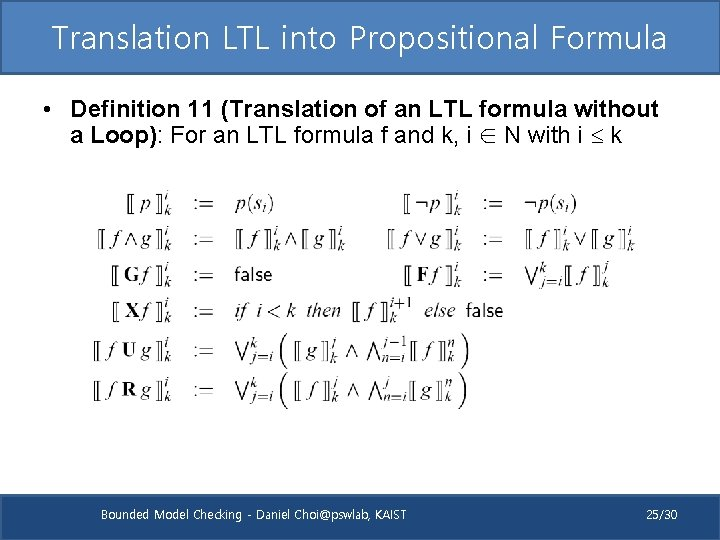Translation LTL into Propositional Formula • Definition 11 (Translation of an LTL formula without a Loop): For an LTL formula f and k, i ∈ N with i k Bounded Model Checking - Daniel [email protected], KAIST 25/30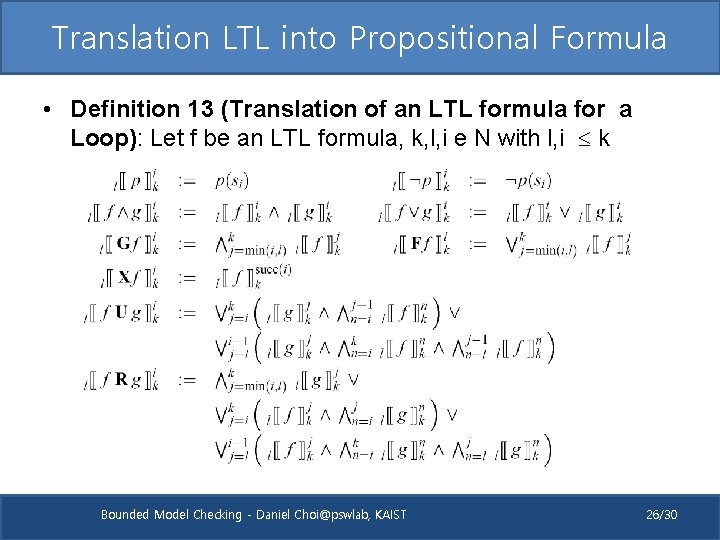Translation LTL into Propositional Formula • Definition 13 (Translation of an LTL formula for a Loop): Let f be an LTL formula, k, l, i e N with l, i k Bounded Model Checking - Daniel [email protected], KAIST 26/30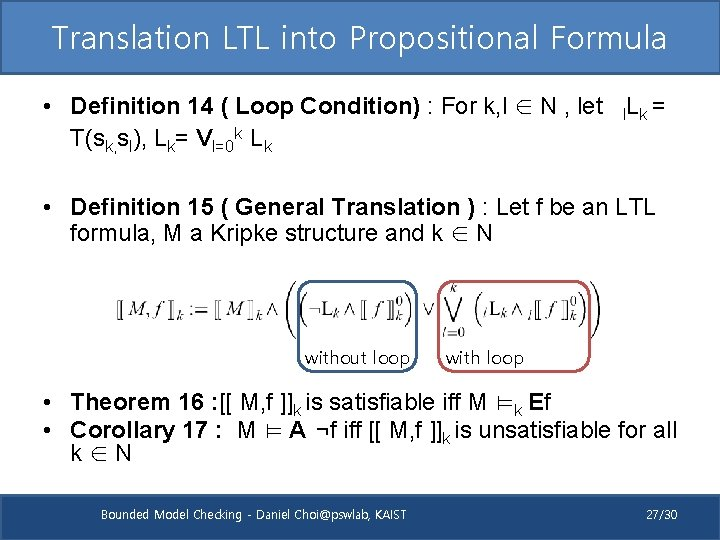Translation LTL into Propositional Formula • Definition 14 ( Loop Condition) : For k, l ∈ N , let l. Lk = T(sk, sl), Lk= Vl=0 k Lk • Definition 15 ( General Translation ) : Let f be an LTL formula, M a Kripke structure and k ∈ N without loop with loop • Theorem 16 : [[ M, f ]]k is satisfiable iff M ⊨k Ef • Corollary 17 : M ⊨ A ¬f iff [[ M, f ]]k is unsatisfiable for all k∈N Bounded Model Checking - Daniel [email protected], KAIST 27/30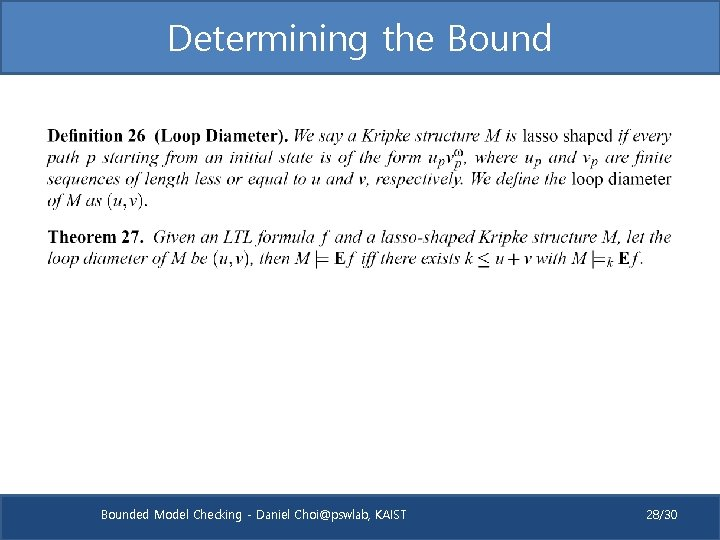Determining the Bounded Model Checking - Daniel [email protected], KAIST 28/30Further Study • CBMC – Making the Most of BMC Counterexamples by Alex Groce, Daniel Koening. In BMC 2004 • This paper introduces counterexample minimization Bounded Model Checking - Daniel [email protected], KAIST 29/30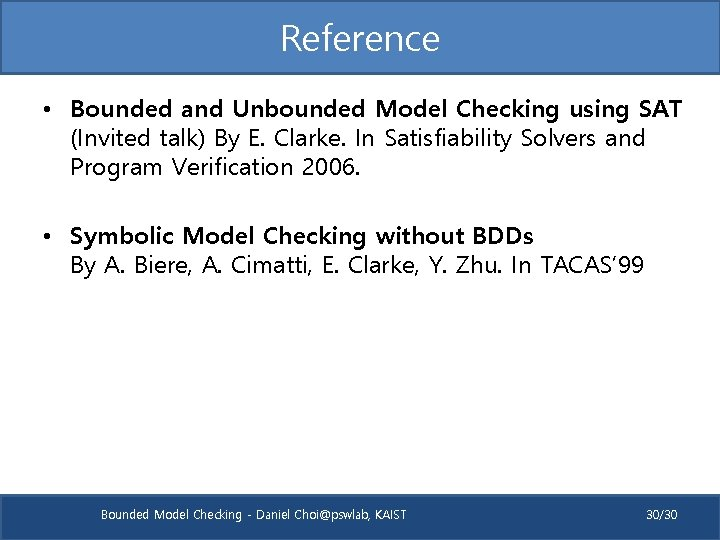Reference • Bounded and Unbounded Model Checking using SAT (Invited talk) By E. Clarke. In Satisfiability Solvers and Program Verification 2006. • Symbolic Model Checking without BDDs By A. Biere, A. Cimatti, E. Clarke, Y. Zhu. In TACAS’ 99 Bounded Model Checking - Daniel [email protected], KAIST 30/30